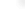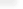Transcendental Roots

Building on a mathematical and algorithmic breakthrough made at Wolfram Research, Mathematica 7 introduces full symbolic coverage of roots of transcendental functions. The systematic treatment of traditional algebraic equations based on polynomials was a central achievement of nineteenth century mathematics. Until now, transcendental equations have mostly been treated on a case-by-case basis. Mathematica 7 for the first time allows systematic treatment of transcendental equations, providing exact representations of roots in a symbolic form suitable for general manipulation, and yielding validated global numerical results to any degree of precision.
• Solutions to arbitrary exp, log equations over the reals.
• Solutions to arbitrary bounded trigonometric equations.
• Solutions to bounded equations involving special functions.
• Solutions to arbitrarily nested transcendental equations.
• General symbolic representation of transcendental equation roots. »
• Fully validated root sets for all equations involving elementary functions.
• Efficient arbitrary-precision numerical evaluation of transcendental roots.
• General algorithm for solving univariate holomorphic equations over bounded regions.
• Provable decidability achieved by interval arithmetic and symbolic methods.
• Full integration with Reduce and other Mathematica functions. »

Related Functions